• python计算macd
千次阅读
2020-12-08 08:47:34

以前在excel里使用表格计算过MACD，上周，花了一天时间查看pandas的帮助和网上其他人的源码，在python3下，自己写了一个计算MACD的源代码。股票数据调用tushare的数据。源码里有详细的注释，可以帮助大家了解如何使用python下的pandas方便快捷计算MACD。

在python3下运行，输入股票代码，比如600642。可以计算所有的股票macd。

——————————————————————————————————–

“””

创建于2017年9月15日 15:11:32

作者：eyeglasses

网站：fx-margin.com

“””

import pandas as pd

import tushare as ts

#定义一个函数get_a_share

def get_a_share(code):

#使用tushare的get_k_data调用A股股票数据

data = ts.get_k_data(code)

#删除列code

data = data.drop(‘code’,axis = 1)

#返回修改后的股票数据

return data

#定义函数，获取macd,导入数据，初始化三个参数

def get_macd_data(data,short=0,long=0,mid=0):

if short==0:

short=12

if long==0:

long=26

if mid==0:

mid=9

#计算短期的ema，使用pandas的ewm得到指数加权的方法，mean方法指定数据用于平均

data[‘sema’]=pd.Series(data[‘close’]).ewm(span=short).mean()

#计算长期的ema，方式同上

data[‘lema’]=pd.Series(data[‘close’]).ewm(span=long).mean()

#填充为na的数据

data.fillna(0,inplace=True)

#计算dif，加入新列data_dif

data[‘data_dif’]=data[‘sema’]-data[‘lema’]

#计算dea

data[‘data_dea’]=pd.Series(data[‘data_dif’]).ewm(span=mid).mean()

#计算macd

data[‘data_macd’]=2*(data[‘data_dif’]-data[‘data_dea’])

#填充为na的数据

data.fillna(0,inplace=True)

#返回data的三个新列

return data[[‘date’,’data_dif’,’data_dea’,’data_macd’]]

#请输入A股股票代码

code = str(input(‘输入A股股票代码:’))

data = get_a_share(code)

macd = get_macd_data(data)

macd

—————————————————————–

注意：在粘贴代码运行的时候，自己把代码缩进调整好。

更多相关内容
• python实现MCDA模型，使用python以类的方式实现MACD。用实例化的方式创建模型使用，其中MACD模型需要实时传入三个数据模型会自动计算实时的MACD值。
• ## python计算macd指标

千次阅读 2019-07-24 15:50:09
移动平滑异同平均线(Moving ...macd计算方法，不求多完美，能对标常用的炒股软件就好了。需要的结果又macd值（红绿柱），diff（快线），dea（慢线） 主要用到talib库talib.MACD方法。 代码实现： diff, dea, my...
移动平滑异同平均线(Moving Average Convergence Divergence，简称MACD指标)策略，被称为“指标之王”，经常使用它，当日要常备在数据库了。

macd计算方法，不求多完美，能对标常用的炒股软件就好了。需要的结果又macd值（红绿柱），diff（快线），dea（慢线）

主要用到talib库talib.MACD方法。

代码实现：

diff, dea, my_macd = talib.MACD(close_all,
fastperiod=12,
slowperiod=26,
signalperiod=9)

其中，closeall是某只股票的一组收盘数据。

下图与 炒股软件对标，基本一致，夫复何求？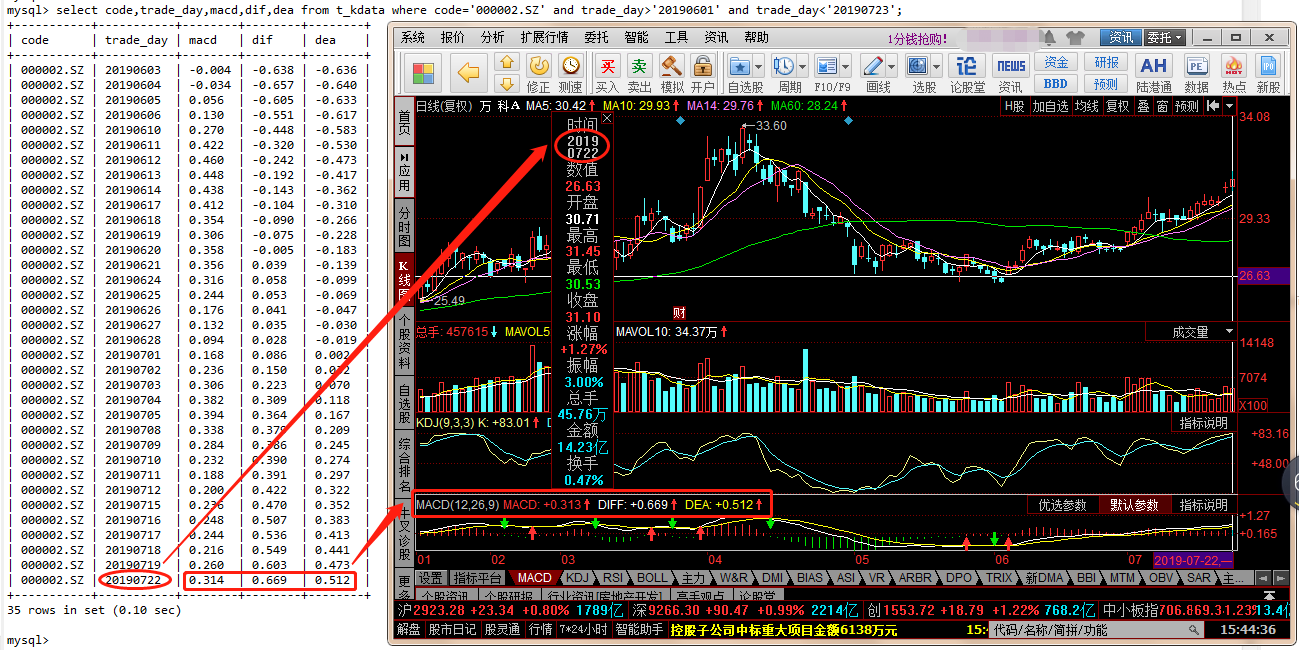展开全文• ## python计算macd的方法

千次阅读 2018-11-17 17:57:03
def calculateEMA ( period ... macd = ( diff - dea ) fast_values = diff slow_values = dea diff_values = macd return fast_values , slow_values , diff_values
def calculateEMA(period, closeArray, emaArray=[]):
length = len(closeArray)
nanCounter = np.count_nonzero(np.isnan(closeArray))
if not emaArray:
emaArray.extend(np.tile([np.nan], (nanCounter + period - 1)))
firstema = np.mean(closeArray[nanCounter:nanCounter + period - 1])
emaArray.append(firstema)
for i in range(nanCounter + period, length):
ema = (2 * closeArray[i] + (period - 1) * emaArray[-1]) / (period + 1)
emaArray.append(ema)
return np.array(emaArray)

def calculateMACD(closeArray, shortPeriod=12, longPeriod=26, signalPeriod=9):
ema12 = calculateEMA(shortPeriod, closeArray, [])
ema26 = calculateEMA(longPeriod, closeArray, [])
diff = ema12 - ema26

dea = calculateEMA(signalPeriod, diff, [])
macd = (diff - dea)

fast_values = diff
slow_values = dea
diff_values = macd

return fast_values, slow_values, diff_values


展开全文• Python计算MACD时的误区 指数函数是重要的基本初等函数之一。一般地，y=a……x函数(a为常数且以a>0，a≠1)叫做指数函数，函数的定义域是 R 。 df['EMA_short'] = df['收盘价_复权'].ewm(span=12, adjust=False)....

# Python计算MACD的误区

指数函数是重要的基本初等函数之一。一般地，y=a^x函数(a为常数且以a>0，a≠1)叫做指数函数，函数的定义域是 R 。

df['EMA_short'] = df['收盘价_复权'].ewm(span=12, adjust=False).mean()


span=12不能实现取出过去12天的数据。
alpha=2/（1+span），span=12意味着df[‘EMA_short’]中每个数都由上一个df[‘EMA_short’]和df[‘收盘价_复权’]生成，上一个df[‘EMA_short’]的权重为11/13，df[‘收盘价_复权’]的权重为2/13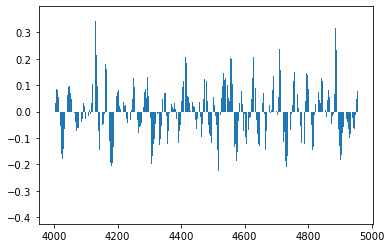同样的数据，上图为上面方法计算的MACD，下图为下面方法计算出的MACD，结果并不相同。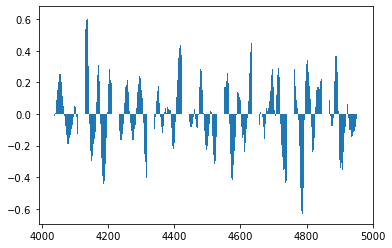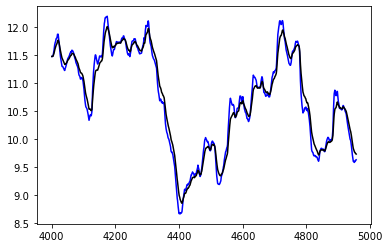同样，上下图是两种不同方法的ema，12日线和36日线。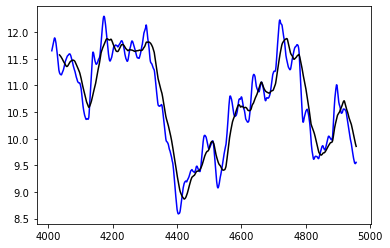正确的代码应该如下：

# 计算MACD
#com等于1相当于权重为1/2，权重为1/(com+1)
#df['EMA_short']中每个数都由上一个df['EMA_short']和df['收盘价_复权']生成，权重各为1/2

df['EMA_short'] = df['EMA_short'].rolling(12).mean()
df['EMA_long'] = df['EMA_long'].rolling(36).mean()
#rolling可以通过滚动，拿出近12日的值，再做平均值，即得到ema
#ewm函数得到的是加权后的值，通过rolling.mean才是ema（指数加权移动平均）


com等于1相当于权重为1/2，权重为1/(com+1)
df[‘EMA_short’]中每个数都由上一个df[‘EMA_short’]和df[‘收盘价_复权’]生成，权重各为1/2
rolling可以通过滚动，拿出近12日的值，再做平均值，即得到ema
ewm函数得到的是加权后的值，通过rolling.mean才是ema（指数加权移动平均）

完整代码：

import pandas as pd
import matplotlib.pyplot as plt

# 读入股票数据

# 计算复权因子
df['复权因子'] = (df['收盘价'] / df['前收盘价']).cumprod()
df['收盘价_复权'] = df['复权因子'] * (df.iloc[-1]['收盘价'] / df.iloc[-1]['复权因子'])

# 计算MACD
#com等于1相当于权重为1/2，权重为1/(com+1)
#df['EMA_short']中每个数都由上一个df['EMA_short']和df['收盘价_复权']生成，权重各为1/2

df['EMA_short'] = df['EMA_short'].rolling(12).mean()
df['EMA_long'] = df['EMA_long'].rolling(36).mean()
#rolling可以通过滚动，拿出近12日的值，再做平均值，即得到ema
#ewm函数得到的是加权后的值，通过rolling.mean才是ema（指数加权移动平均）

plt.figure()
plt.plot(df['EMA_short'],'b')
plt.plot(df['EMA_long'],'k')

df['DIF'] = df['EMA_short'] - df['EMA_long']
df['MACD'] = (df['DIF'] - df['DEA']) * 2
plt.figure()
plt.bar(df['MACD'].index,df['MACD'].values)

展开全文算法
• 作者：Cameron Shadmehry编译：Florence Wong – AICUG本文系AICUG翻译原创...图说：颜色表示MACD预测价格走势的准确性，而大小表示记录的交叉量。MACD技术指标的准确性非常不稳定，取决于所观察到的库存，它经常跌...
• Q1：python计算macd等技术指标，有什么包吗有的，包的链接如下：https://github.com/lovelylain/pyctp/tree/master/example/ctp/talibQ2：通达信什么时候支持python量化交易1、一个强大的N维数组对象Array；...
• PythonMACD画图范例 代码非常简单： ‘’‘backtest start: 2020-01-28 00:00:00 end: 2020-02-26 00:00:00 period: 1d exchanges: [{“eid”:“OKEX”,“currency”:“BTC_USDT”}] ‘’’ ChartCfg = { ‘__...
• ## python 实现股票MACD计算

万次阅读 热门讨论 2018-09-02 19:58:07
首先普及基本知识，就是MACD计算公式： 3个参数（这3个参数可以根据实际情况自己设定，默认为12，26和9）：（12）日快速移动平均线，（26）日慢速移动平均，（9）日移动平均 EMA（12）= 前一日EMA（12）×11/13＋...
• python macd算法Machine Learning trading works just fine for large hedge funds that make thousands of trades per day. However, private traders just don’t have the facilities to run machine learning ...算法 人工智能 机器学习 java
• 正如下面的MACD顶背离技术形态，仔细观察价格与MACD两者的走势。 如下图所示： 从上图中可以看到，价格的波峰一个比一个高，而MACD指标的波峰一浪比一浪低。这就是MACD顶背离！ 而MACD底背离，如下图所示: ...
• 1 计算公式 MACD指标主要有三个：MACD、DIF、DEA。MACD是从双指数移动平均线发展而来的，由快的指数移动平均线（EMA12）减去慢的指数移动平均线（EMA26）得到快线DIF，再用2×（快线DIF-DIF的9日加机器学习
• 本文就全面的讲解一下MACD指标，用Python和全量的历史数据，看看它的表现到底如何。 01 什么是MACD 不管你是交易A股、美股、期货还是数字货币，大部分的行情软件都会将MACD指标默认显示在指标的前几位。 ...
• talib_macd.py # -*- coding: utf-8 -*- import os, sys import tushare as ts import pandas as pd import matplotlib.pyplot as plt import numpy as np import talib if len(sys.argv) ==2: code = sys..
• 邢不行的系列帖子“量化小讲堂”，通过实际案例教初学者使用python进行量化投资，了解行业研究方向，希望能对大家有帮助 ​ ​ 如果要说哪个技术指标知道的人最多，那估计非MACD莫属了。 因为不管你是炒A...
• python代码: import talib as ta # m is macd # s is signal # h is histogram m,s,h = ta.MACD(data, fastperiod=20, slowperiod=40, signalperiod=10) 我不怀疑交易软件是错误的,考虑到python给出了相同的结果,我...
• python 量化交易：MACD指标双金叉形态识别： df 是pandas的Dataframe，基础数据原来是 tushare 中的股票交易数据，MACD指标是用 talib 库计算得来： df = df.sort_values(by='trade_date', ascending=True) # ...
• ## python实现MACD均线择时策略

千次阅读 多人点赞 2020-11-01 20:48:33
MACD（Moving Average Convergence and Divergence)即指数平滑移动平均线，由Geral Appel 于1970年提出，属于大势趋势类指标，它由***长期慢速均线DEA，短期快线的DIF，红色能量柱（多头），绿色能量柱（空头）、0轴...量化投资
• MACD指标定义 MACD称为异同移动平均线，是从双指数移动平均线发展而来的，由快的指数移动平均线（EMA12）减去慢的指数移动平均线（EMA26）得到快线DIF，再用2×...MACD计算两条不同速度（长期与中期）的异同移动平开发语言 后端
• 如果您熟悉python编程，则可以检查 ，它提供了强大的统计指标支持，并由和支持。 的性能比JavaScript库高很多倍，并且可以由机器学习程序直接使用。麦克德金融科技工具来计算MACD是“移动平均汇聚/散度”的缩写...JavaScript
• 最近很多朋友使用老虎的开放API时， 都会咨询我们获取 MACD、KDJ 等技术指标的方法。开放API没有提供计算好的技术指标数据， 因为这类指标的计算方法比较简单。这里做一个简单的示例，供大家参考：首先需要安装ta-...
• 缠论辅助同花顺面积公式原同花顺只有面积无数值显示，修改方法：打开同花顺，工具→公式管理→趋势指标→MACD→修改→把第一种或第二种的数据复制掩盖原有数据→确定，大功告成，面积数出来了。第一种：DIF:EMA...
• talib-macd-example 在Python中使用matplotlib使用 （在Python 2.7中）呈现指标的示例。 这段代码是对名为 的用户在上的问题的解答。 他的问题可以在找到。...